Test: Polynomials- Assertion & Reason Type Questions- 2

# Test: Polynomials- Assertion & Reason Type Questions- 2

Test Description

## 14 Questions MCQ Test Advance Learner Course: Mathematics (Maths) Class 8 | Test: Polynomials- Assertion & Reason Type Questions- 2

Test: Polynomials- Assertion & Reason Type Questions- 2 for Class 9 2022 is part of Advance Learner Course: Mathematics (Maths) Class 8 preparation. The Test: Polynomials- Assertion & Reason Type Questions- 2 questions and answers have been prepared according to the Class 9 exam syllabus.The Test: Polynomials- Assertion & Reason Type Questions- 2 MCQs are made for Class 9 2022 Exam. Find important definitions, questions, notes, meanings, examples, exercises, MCQs and online tests for Test: Polynomials- Assertion & Reason Type Questions- 2 below.
Solutions of Test: Polynomials- Assertion & Reason Type Questions- 2 questions in English are available as part of our Advance Learner Course: Mathematics (Maths) Class 8 for Class 9 & Test: Polynomials- Assertion & Reason Type Questions- 2 solutions in Hindi for Advance Learner Course: Mathematics (Maths) Class 8 course. Download more important topics, notes, lectures and mock test series for Class 9 Exam by signing up for free. Attempt Test: Polynomials- Assertion & Reason Type Questions- 2 | 14 questions in 30 minutes | Mock test for Class 9 preparation | Free important questions MCQ to study Advance Learner Course: Mathematics (Maths) Class 8 for Class 9 Exam | Download free PDF with solutions
 1 Crore+ students have signed up on EduRev. Have you?
Test: Polynomials- Assertion & Reason Type Questions- 2 - Question 1

### Direction: In the following questions, a statement of assertion (A) is followed by a statement of reason (R). Mark the correct choice as:Assertion : The sum and product of the zeros of a quadratic polynomial are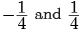respectively.Then the quadratic polynomial is 4x2 +x+1.Reason : The quadratic polynomial whose sum and product of zeros are given is x2-(sum of zeros). x + product of zeros.

Detailed Solution for Test: Polynomials- Assertion & Reason Type Questions- 2 - Question 1

Sum of zeros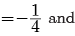product of zeros = 1/4

Quadratic polynomial be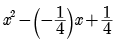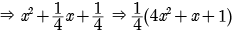Quadratic polynomial be 4x2 +x + 1. So, both A and R are correct and R explains A.

Test: Polynomials- Assertion & Reason Type Questions- 2 - Question 2

### Direction: In the following questions, a statement of assertion (A) is followed by a statement of reason (R). Mark the correct choice as:Assertion : If (x + 1) is a factor of f(x) = x2 + ax + 2, then a = - 3.Reason : If (x - a) is a factor of p (x), if p (a) = 0.

Detailed Solution for Test: Polynomials- Assertion & Reason Type Questions- 2 - Question 2 If p (a) = 0, then x-a is a factor of that polynomial p(x). x - a would be the factor of the polynomial if the r(x) i.e. remainder is 0.
Test: Polynomials- Assertion & Reason Type Questions- 2 - Question 3

### Direction: In the following questions, a statement of assertion (A) is followed by a statement of reason (R). Mark the correct choice as:Assertion : If both zeros of the quadratic polynomial x2 - 2kx + 2 are equal in magnitude but opposite in sign then value of k is 1/2.Reason : Sum of zeros of a quadratic polynomial ax2 + bx + c is b/a

Detailed Solution for Test: Polynomials- Assertion & Reason Type Questions- 2 - Question 3 As the polynomial is x2 - 2kx + 2 and its zeros are equal but opposition sign, sum of zeroes must be zero.

sum of zeros = 0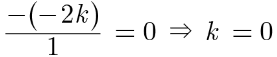Assertion (A) is false but reason (R) is true.

Thus (d) is correct option.

Test: Polynomials- Assertion & Reason Type Questions- 2 - Question 4

Direction: In the following questions, a statement of assertion (A) is followed by a statement of reason (R). Mark the correct choice as:

Assertion : x3 + x has only one real zero.

Reason : A polynomial of nth degree must have n real zeroes.

Detailed Solution for Test: Polynomials- Assertion & Reason Type Questions- 2 - Question 4 Reason is false [a polynomial of nth degree has at most x zeroes.]

Again, x3 +x = x(x2 + 1)

which has only one real zero

(x = 0) [x2 + 1 ≠ 0 for all x ε R]

Assertion is true.

Test: Polynomials- Assertion & Reason Type Questions- 2 - Question 5

Direction: In the following questions, a statement of assertion (A) is followed by a statement of reason (R). Mark the correct choice as:

Assertion : The remainder when p(x) = x3 - 6x2 + 2x - 4 is divided by (3x - 1) is -107/27.

Reason : If a polynomial p(x) is divided by ax - b , the remainder is the value of p(x) at x = b/a.

Detailed Solution for Test: Polynomials- Assertion & Reason Type Questions- 2 - Question 5

p(x) = x3 - 6x2 + 2x - 4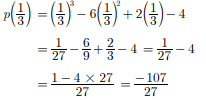Test: Polynomials- Assertion & Reason Type Questions- 2 - Question 6

Direction: In the following questions, a statement of assertion (A) is followed by a statement of reason (R). Mark the correct choice as:

Assertion : P(x) = 14x3 - 2x2 + 8x4 + 7x - 8 is a polynomial of degree 3.

Reason : The highest power of x in the polynomial p(x) is the degree of the polynomial.

Detailed Solution for Test: Polynomials- Assertion & Reason Type Questions- 2 - Question 6 The highest power of x in the polynomial p(x)

= 14x3 - 2x2 + 8x4 + 7x - 8 is 4.

Degree of p(x) is 4. So, A is incorrect but R is correct.

Test: Polynomials- Assertion & Reason Type Questions- 2 - Question 7

Direction: In the following questions, a statement of assertion (A) is followed by a statement of reason (R). Mark the correct choice as:

Assertion : (x + 2) is a factor of x3 + 3x2 + 5x + 6 and of 2x + 4.

Reason : If p(x) be a polynomial of degree greater than or equal to one, then (x - a) is a factor of p(x), if p(a) = 0

Detailed Solution for Test: Polynomials- Assertion & Reason Type Questions- 2 - Question 7 p(x) = x3 + 3x2 + 5x + 6

g(x) = x + 2

x = -2

put x = -2 in p(x)

p(-2) = (-2)3 + 3(-2)2 + 5(-2) + 6

= -8 + 12 - 10 + 6

= 0

since p(x) is zero

X + 2 is a factor of x3 + 3x2 + 5x + 6

Checking

let x = -2 q(x) = 2x + 4

q(-2) = 2(-2) + 4

= -4 + 4

= 0

since reminder is zero

x + 2 is a factor of 2x + 4

Test: Polynomials- Assertion & Reason Type Questions- 2 - Question 8

Direction: In the following questions, a statement of assertion (A) is followed by a statement of reason (R). Mark the correct choice as:

Assertion : If one zero of poly-nominal p(x) = (k2 + 4)x2 + 13x + 4k is reciprocal of other, then k = 2.

Reason : If (x - α) is a factor of p(x), then p(α) = 0

i.e. α is a zero of p(x).

Detailed Solution for Test: Polynomials- Assertion & Reason Type Questions- 2 - Question 8 Reason is true.

Let α, 1/α be the zeroes of p(x), then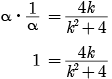k2 - 4k + 4 = 0

(k - 2)2 = 0

k = 2

Assertion is true Since, Reason is not correct for Assertion.

Test: Polynomials- Assertion & Reason Type Questions- 2 - Question 9

Direction: In the following questions, a statement of assertion (A) is followed by a statement of reason (R). Mark the correct choice as:

Assertion : The expression 3x4 - 4x3/2 + x2 = 2 is not a polynomial because the term -4x3/2 contains a rational power of x.

Reason : The highest exponent in various terms of an algebraic expression in one variable is called its degree.

Detailed Solution for Test: Polynomials- Assertion & Reason Type Questions- 2 - Question 9 A rational function is defined as the ratio of two real polynomials with the condition that the polynomial in the denominator is not a zero polynomial. For any function, the x -intercepts are x -values for which the function has a value of zero: f(x) = 0, f (x) = 0.
Test: Polynomials- Assertion & Reason Type Questions- 2 - Question 10

Direction: In the following questions, a statement of assertion (A) is followed by a statement of reason (R). Mark the correct choice as:

Assertion : x2 + 4x + 5 has two zeroes.

Reason : A quadratic polynomial can have at the most two zeroes.

Detailed Solution for Test: Polynomials- Assertion & Reason Type Questions- 2 - Question 10

Assertion

Consider the given polynomial x2 + 4x + 5

Because the degree of the polynomial is 2. It is a quadratic polynomial.

We know that the quadratic polynomial has at the most two zeroes.

∴ x2 + 4x + 5 has two zeroes.

∴ Assertion is true.

Reason Clearly, Reason is true.

Since both the Assertion and Reason are true and Reason is a correct explanation of Assertion.

Test: Polynomials- Assertion & Reason Type Questions- 2 - Question 11

Direction: In the following questions, a statement of assertion (A) is followed by a statement of reason (R). Mark the correct choice as:

Assertion : 3x2 + x - 1 = (x + 1) (3x - 2) + 1.

Reason : If p(x) and g(x) are two polynomials such that degree of p(x) ≥ degree of g(x) and g(x) ≥ 0 then we can find polynomials q(x) and r(x) such that p(x) = g(x) q(x) + r (x), where r(x) = 0 of degree of r(x) < degree of g(x).

Detailed Solution for Test: Polynomials- Assertion & Reason Type Questions- 2 - Question 11 Let p(x) and g(x) be two polynomials

If g(x) is any polynomial then it can divide p(x) by q(x) where 0 < q(x) and may get a remainder say r(x).

If g(x) perfectly divides p(x) by q(x), then r(x) = 0.

It is obvious that deg r(x) < deg g(x).

∴ we can find polynomial q(x) and r(x) such that

p(x) = q(x)q(x) + r(x), where r(x) = 0 or deg r(x) < deg g(x)

Test: Polynomials- Assertion & Reason Type Questions- 2 - Question 12

Direction: In the following questions, a statement of assertion (A) is followed by a statement of reason (R). Mark the correct choice as:

Assertion : (x + 2) and (x - 1) are factors of the polynomial x4 + x3 + 2x2 + 4x - 8.

Reason : For a polynomial p(x) of degree ≥ 1, x - a is a factor of the polynomial p(x) if and only if p(a) ≥ 1.

Detailed Solution for Test: Polynomials- Assertion & Reason Type Questions- 2 - Question 12

p(x) = x4 + x3 + 2x2 + 4x - 8

p(-2) = (-2)4 + (-2)3 + 2(-2)2 + 4 (-2) - 8

= 16 - 8 + 8 - 8 - 8 = 0

So, (x + 2) is a factor of p(x).

p(1) = (1)4 + (1)3 + 2(1)2 + 4(1) - 8

= 1 + 1 + 2 + 4 - 8 = 0

(x - 1) is a factor of p(x).

Test: Polynomials- Assertion & Reason Type Questions- 2 - Question 13

Direction: In the following questions, a statement of assertion (A) is followed by a statement of reason (R). Mark the correct choice as:

Assertion : (2 - √3) is one zero of the quadratic polynomial then other zero will be (2 + √3).

Reason : Irrational zeros (roots) always occurs in pairs.

Detailed Solution for Test: Polynomials- Assertion & Reason Type Questions- 2 - Question 13 As irrational roots/zeros always occurs in pairs therefore, when one zero is (2 - √3) then other will be (2 + √3) . So, both A and R are correct and R explains A.
Test: Polynomials- Assertion & Reason Type Questions- 2 - Question 14

Direction: In the following questions, a statement of assertion (A) is followed by a statement of reason (R). Mark the correct choice as:

Assertion : If f(x) = 3x7 - 4x6 + x + 9 is a polynomial, then its degree is 7.

Reason : Degree of a polynomial is the highest power of the variable in it.

Detailed Solution for Test: Polynomials- Assertion & Reason Type Questions- 2 - Question 14 The degree of a polynomial function is the highest power of the variable that occurs in a polynomial. The term containing the highest power of the variable is called the leading term. The coefficient of the leading term is called the leading coefficient.

## Advance Learner Course: Mathematics (Maths) Class 8

13 videos|61 docs|24 tests
 Use Code STAYHOME200 and get INR 200 additional OFF Use Coupon Code
Information about Test: Polynomials- Assertion & Reason Type Questions- 2 Page
In this test you can find the Exam questions for Test: Polynomials- Assertion & Reason Type Questions- 2 solved & explained in the simplest way possible. Besides giving Questions and answers for Test: Polynomials- Assertion & Reason Type Questions- 2, EduRev gives you an ample number of Online tests for practice

## Advance Learner Course: Mathematics (Maths) Class 8

13 videos|61 docs|24 tests